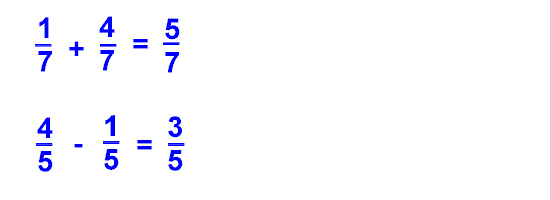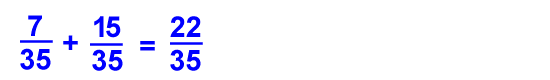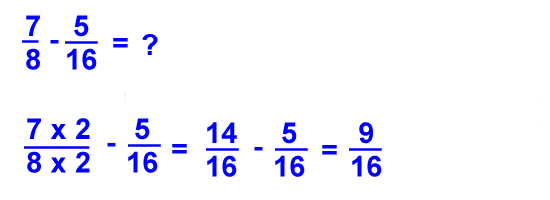Parents and Teachers: Support Ducksters by following us onor.

Kids Math

Adding and subtracting fractions may seem tricky at first, but if you follow a few simple steps and work a lot of practice problems, you will have the hang of it in no time.

Here are some steps to follow:
• Check to see if the fractions have the same denominator.
• If they don't have the same denominator, then convert them to equivalent fractions with the same denominator.
• Once they have the same denominator, add or subtract the numbers in the numerator.
Note: The denominator may have changed when you converted the fractions to the same common denominator.

Simple Example

A simple example is when the denominators are already the same:Since the denominators are the same in each question, you just add or subtract the numerators to get the answers.

Harder Example

Here we will try a problem where the denominators are not the same.As you can see, these fractions do not have the same denominator. Before we can add the fractions together, we must first create equivalent fractions that have common denominators.

Find the Common Denominator

To find a common denominator, we must multiply each fraction by the other fraction's denominator (the one the bottom). If we multiply both the top and the bottom of the fraction by the same number, its just like multiplying it by 1, so the value of the fraction stays the same. See the example below:Now that the denominators are the same, you can add the numerators and put the answer over the same denominator.

Subtracting Fractions Example

Here is an example of subtracting fractions where only one denominator needs to be changed:Sometimes the answer will need to be reduced. Here is an example:The initial answer after adding the numerators was 10/15, however this fraction can be further reduced to 2/3 as shown in the last step.

Tips for Adding and Subtracting Fractions
• Always make sure that the denominators are the same before you add or subtract.
• If you multiply the top and the bottom of a fraction by the same number, the value stays the same.
• Be sure to practice converting fractions to common denominators. This is the hardest part of adding and subtracting fractions.
• You may need to simplify your answer after you are done adding and subtracting. Sometimes the answer can be reduced even though the original fractions could not be reduced.
• The same process is used for both adding and subtracting, if you can add fractions, you can subtract them.
• If there are mixed numbers that you are adding or subtracting be sure to convert them to improper fractions before you start the process.

Back to Kids Math

Back to Kids StudyFollow us onor